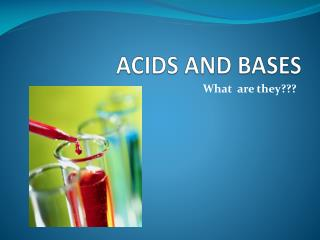# ACIDS and BASES - PowerPoint PPT PresentationDownload PresentationACIDS and BASES

ACIDS and BASESDownload Presentation## ACIDS and BASES

- - - - - - - - - - - - - - - - - - - - - - - - - - - E N D - - - - - - - - - - - - - - - - - - - - - - - - - - -
##### Presentation Transcript

1. ACIDS and BASES What are they???

2. What are they? • Acids and bases are very common. • Many familiar compounds are acids or bases. • Acids are Sour , Bases are Bitter • Classification as acids or bases is based on chemical composition. (what chemicals they are made of) • Acids and bases can be very dangerous! • Acids are Corrosive(BURN skin and insides!). • Bases are Caustic (rashes, burning) • The strength of acids and bases in measured on the pH SCALE

3. pH SCALE • 0 1 2 3 4 5 6 78 9 10 11 12 13 14 • pH below 7 = acidic pH above 7= basic pH7 = neutral

4. What does the pH scale mean? • Each decrease of 1 on the pH scale indicates 10X more acidic • For example, pH 4 is ten times more acidic than pH 5 • pH 3 (Grapes) is 1000X more acidic than pH6 (milk)

5. How do we determine the pH?aka. How do we determine if something is an Acid or a base? • The pH of acids and bases cannot be determined by sight, instead, pH is measured by 2 other methods: 1) Indicators –chemicals that change colour depending on which pH they are placed in. ∙The most common type of indicator is Litmus, used on litmus paper. There are two colours of litmus paper: Blue and Red • If litmus paper turns/stays Blue = pH above 7 = Base • If Litmus paper turns/stays Red = pH below 7 = Acid

6. Liquid Chemicals are another common type of indicator. These chemicals change colour at different pH’s • Eg. Bromothymol Blue is an indicator used to test for pH 6 - 7.6, • Eg. Phenolphthalein is an indicator used to test for pH 8.2 – 10 • Many natural sources, such as beets and cabbage, are also indicators

7. The second method of determining pH 2) A pH meter is another method of measuring pH ∙ Both Acids and Bases conduct electricity when dissolved in water. A pH meter measures the electrical conductivity of the solution – it uses electric probes that you place in a solution and it measures how electricity is conducted in the solution.

8. There is 1 easy way to tell if something is an Acid or a Base… • If you know the chemical formula of your unknown chemical – you can tell if it is an acid or a base. • Acids are often written with subscript (aq) = aquatic = water • Acids conduct electricity because they release hydrogen ions, H+(aq) • The chemical formula of an acid usually starts with Hydrogen (H-). • Acids with a carbon usually have the C written first.

9. Examples of some Acids • HCl(aq) = hydrochloric acid, • HNO3(aq) = nitric acid • CH3COOH(aq) = acetic acid • H2SO4 (aq) = Sulphuric acid

10. Bases, like acids, are often written with subscript (aq) = aquatic = water • Bases release hydroxide ions OH–(aq) • The chemical formula of a base usually ends with hydroxide (-OH). • Bases can be harmless or very caustic • Examples of common bases • NaOH(aq) – Sodium Hydroxide • Mg(OH)2(aq) – Magnesium Hydroxide • Ca(OH)2(aq) – Calcium Hydroxide • NH4OH(aq) – Ammonium Hydroxide